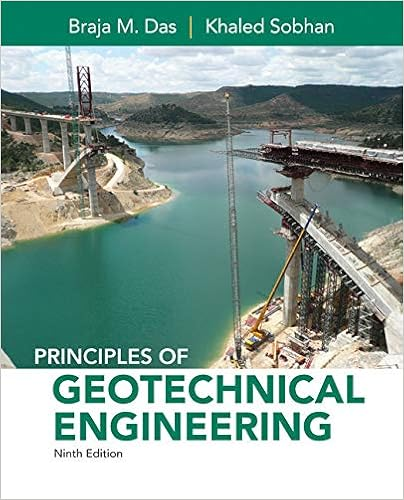# G let t be the first positive value in 01 where the

• Homework Help
• 15
• 75% (16) 12 out of 16 people found this document helpful

This preview shows page 9 - 13 out of 15 pages.

##### We have textbook solutions for you!
The document you are viewing contains questions related to this textbook.The document you are viewing contains questions related to this textbook.
Chapter 15 / Exercise 15.16
Principles of Geotechnical Engineering
Das/SobhanExpert Verified
(g) Let t*be the first positive value in [0,1] where the curve crosses the y-axis. For what value of bis the tangent line to the curve at P(t*)parallel to y = -5 x:
Viewing Saved Work Revert to Last Response9. 6/6 points | Previous Answers My Notes Question PartPointsSubmissions Used1234561/1 1/1 1/1 1/1 1/1 1/11/5 2/5 1/5 4/5 4/5 1/5Total6/6A major league baseball pitcher throws a pitch that follows these parametric equations:x(t) = 142y(t) = –16t2+ 4t + 5.The time units are seconds and the distance units are feet. The distance between the location of the pitcherand homeplate (where the batter stands) is 60.5 feet. Give EXACT answers, unless instructed otherwise. (a) Calculate the horizontal velocity of the baseball at time t; this is the function x'(t)t=
(b) What is the horizontal velocity of the baseball when it passes over homeplate?
Practice Another Version
##### We have textbook solutions for you!
The document you are viewing contains questions related to this textbook.The document you are viewing contains questions related to this textbook.
Chapter 15 / Exercise 15.16
Principles of Geotechnical Engineering
Das/SobhanExpert Verified
ft/sec (c) What is the vertical velocity of the baseball at time t; this is the function y'(t)=
(d) Recall that the speed of the baseball at time tis s(t)=√ [x'(t)]2 + [y' (t)]2 ft/secWhat is the speed of the baseball (in mph) when it passes over homeplate? .
(e) At what time does the baseball hit the ground, assuming the batter and catcher miss the ball?
(f) What is the magnitudeof the angle at which the baseball hits the ground?
Viewing Saved Work Revert to Last Response10. 12/12 points | Previous Answers My Notes Question PartPointsSubmissions Used1234567891011121/1 1/1 1/1 1/1 1/1 1/1 1/1 1/1 1/1 1/1 1/1 1/11/5 1/5 1/5 1/5 1/5 2/5 1/5 1/5 1/5 2/5 1/5 2/5Total12/12Practice Another Version
Consider an object moving in the plane whose location at time tseconds is given by the parametric equations:x(t)=5cos(πt)y(t)=2sin(πt). Assume the distance units in the plane are meters. (a) The object is moving around an ellipse (as in the previous problem) with equation:x2a2+ y2b2=1 where a= \$\$5 and b= \$\$2 .(b) The location of the object at time t=1/3 seconds is ( \$\$2.5 , \$\$1.732 ).(c) The horizontal velocity of the object at time t is x ' (t)= \$\$−5π·sin(π·t) m/s.(d) The horizontal velocity of the object at time t=1/3 seconds is
•••